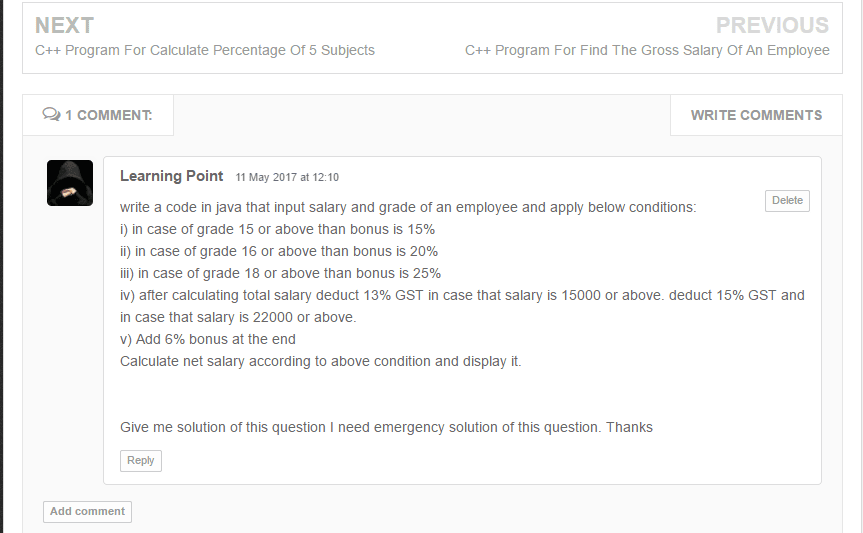# Employee Salary Program in Java [Calculate Employee Bonus]

Program to calculate bonuses for different departments using method overriding. Employee salary program in java. Employee bonus calculation in java. Please see this is a requested problem from Foreign Country. See you can also request any problem regarding programming. So don't forget to share thanks, learning point (Foreign Country) writing for us.

Write a code in java that inputs the salary and grade of an employee and apply conditions.

• in case of grade 15 or above then the bonus is 15%
• in case of grade 16 or above then the bonus is 20%
• in case of grade 18 or above then the bonus is 25%
• after calculating the total salary deduct 13% GST in case that salary is 15000 or above. Deduct 15% GST in case that salary is 22000 or above.
• add a 6% Employee Bonus at the end

Calculate net salary according to the above condition and display it. Employee salary program in java.So according to the first three conditions in the problem, I use an if-else statement for an Employee Bonus see below.

``````if (grade == 15) {
bonus = (basic_salary * 15) / 100;
bonus = (basic_salary * 20) / 100;
} else if (grade >= 18) {
bonus = (basic_salary * 25) / 100;
}``````

After that, we get a basic salary + bonus. Now according to GST(Goods and Service Tax), conditions calculate a remaining employee salary program in java after all calculations 6% Employee bonus.

``````if (basic_salary >= 15000 && basic_salary = 22000) {
gst = (basic_salary * 15) / 100;

basic_salary -= gst;

bonus1 = (basic_salary * 6) / 100;

basic_salary += bonus1;

} else {
bonus1 = (basic_salary * 6) / 100;

basic_salary += bonus1;
}``````

At the end print the Salary.

Example: Let's assume the employee salary program in java is 20,000 and grade is 15 then calculate an employee's salary with Employee Bonus.

20,000 salary and the 15-grade bonus is 15% then bonus = 3000.

• Now the total salary is 20,000+3000=23,000
• Now salary is greater than 22,000 then 15% GST, so GST = 3450.
• Now the total salary is 23,000 - 3450 = 19550.
• At the end 6% bonus then bonus = 1173
• The gross salary of an employee is =19500+1173=20723.

Gross salary = 20723.

Try This Program to Calculate the Gross Salary of an Employee in Java

## Employee Salary Program in Java

``````import java.util.Scanner;
import java.lang.*;

/* Employee Salary Program in Java or Employee Bonus Calculation in Java*/

class employeesalary {

public static void main(String args[]) {
float basic_salary, bonus1 = 0, bonus = 0, gst;

Scanner scan = new Scanner(System.in);

System.out.println("Enter the Employee Basic Salary: ");
basic_salary = scan.nextFloat();

System.out.println("\nEnter the Employee Bonus: ");

bonus = (basic_salary * 15) / 100;
bonus = (basic_salary * 20) / 100;
} else if (grade >= 18) {
bonus = (basic_salary * 25) / 100;
}

basic_salary += bonus;

if (basic_salary >= 15000 && basic_salary = 22000) {
gst = (basic_salary * 15) / 100;

basic_salary -= gst;

bonus1 = (basic_salary * 6) / 100;

basic_salary += bonus1;
} else {
bonus1 = (basic_salary * 6) / 100;

basic_salary += bonus1;
}

System.out.println("\nGross Employee Salary is: " + basic_salary);
}
}``````

### Similar to Employees Salary

Previous Post
Next Post

#### post written by: Ghanendra Yadav

Hi, I’m Ghanendra Yadav, SEO Expert, Professional Blogger, Programmer, and UI Developer. Get a Solution of More Than 500+ Programming Problems, and Practice All Programs in C, C++, and Java Languages. Get a Competitive Website Solution also Ie. Hackerrank Solutions and Geeksforgeeks Solutions. If You Are Interested to Learn a C Programming Language and You Don't Have Experience in Any Programming, You Should Start with a C Programming Language, Read: List of Format Specifiers in C.